Related Articles
DBScan Clustering in R Programming
• Last Updated : 02 Jul, 2020

Density-Based Clustering of Applications with Noise(DBScan) is an Unsupervised learning Non-linear algorithm. It does use the idea of density reachability and density connectivity. The data is partitioned into groups with similar characteristics or clusters but it does not require specifying the number of those groups in advance. A cluster is defined as a maximum set of densely connected points. It discovers clusters of arbitrary shapes in spatial databases with noise.

#### Theory

In DBScan clustering, dependence on distance-curve of dimensionality is more. The algorithm is as follows:

1. Randomly select a point p.
2. Retrieve all the points that are density reachable from p with regard to Maximum radius of the neighbourhood(EPS) and minimum number of points within eps neighborhood(Min Pts).
3. If the number of points in the neighborhood is more than Min Pts then p is a core point.
4. For p core points, a cluster is formed. If p is not a core point, then mark it as a noise/outlier and move to the next point.
5. Continue the process until all the points have been processed.

DBScan clustering is insensitive to order.

#### The Dataset

`Iris` dataset consists of 50 samples from each of 3 species of Iris(Iris setosa, Iris virginica, Iris versicolor) and a multivariate dataset introduced by British statistician and biologist Ronald Fisher in his 1936 paper The use of multiple measurements in taxonomic problems. Four features were measured from each sample i.e length and width of the sepals and petals and based on the combination of these four features, Fisher developed a linear discriminant model to distinguish the species from each other.

 `# Loading data``data(iris)``  ` `# Structure ``str``(iris)`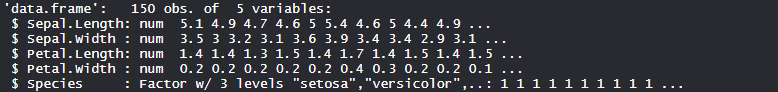#### Performing DBScan on Dataset

Using the DBScan Clustering algorithm on the dataset which includes 11 persons and 6 variables or attributes

 `# Installing Packages``install.packages(``"fpc"``)`` ` `# Loading package``library(fpc)`` ` `# Remove label form dataset``iris_1 <``-` `iris[``-``5``]`` ` `# Fitting DBScan clustering Model ``# to training dataset``set``.seed(``220``)  ``# Setting seed``Dbscan_cl <``-` `dbscan(iris_1, eps ``=` `0.45``, MinPts ``=` `5``)``Dbscan_cl`` ` `# Checking cluster``Dbscan_cl\$cluster`` ` `# Table``table(Dbscan_cl\$cluster, iris\$Species)`` ` `# Plotting Cluster``plot(Dbscan_cl, iris_1, main ``=` `"DBScan"``)``plot(Dbscan_cl, iris_1, main ``=` `"Petal Width vs Sepal Length"``)`

#### Output:

• Model dbscan_cl: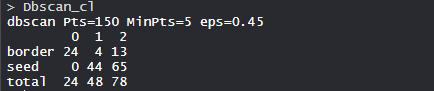In the model, there are 150 Pts with Minimum points are 5 and eps is 0.5.

• Cluster identification: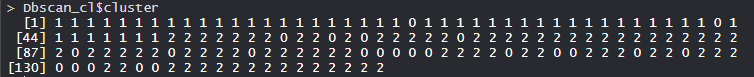The clusters in the model are shown.

• Plotting Cluster: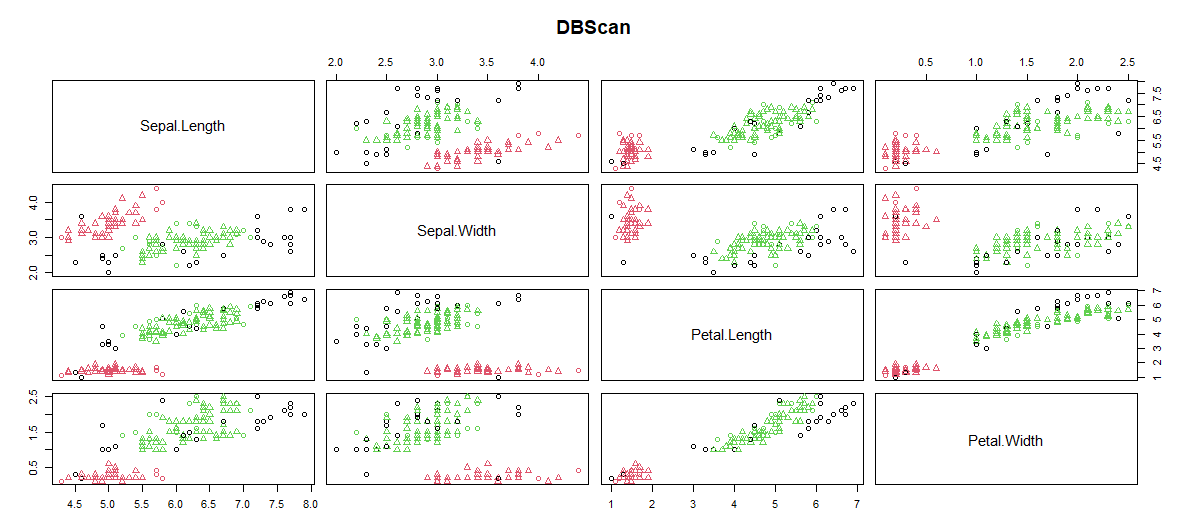DBScan cluster is plotted with Sepal.Length, Sepal.Width, Petal.Length, Petal.Width.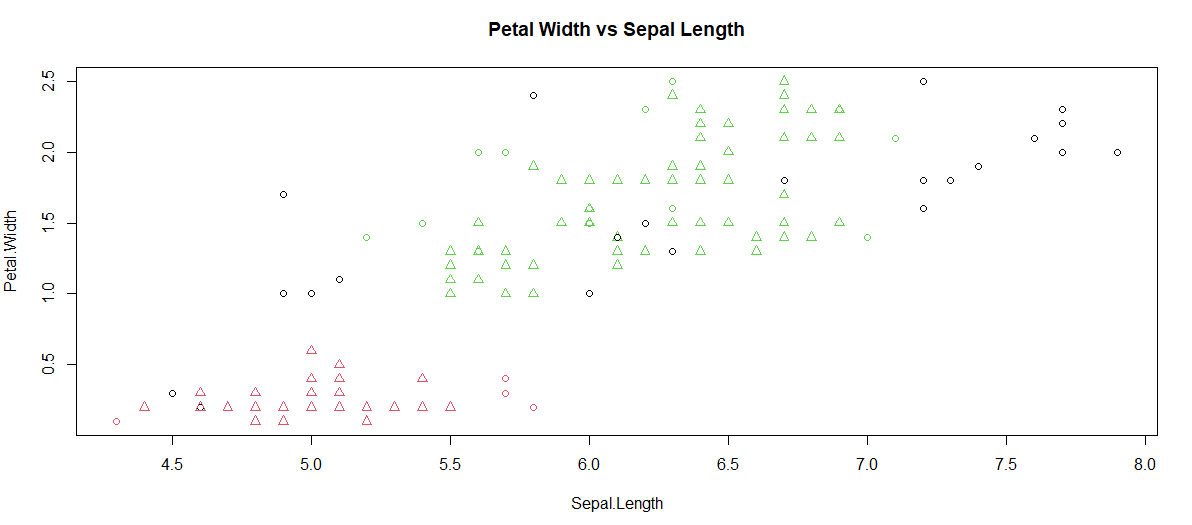The plot is plotted between Petal.Width & Sepal.Length.

So, the DBScan clustering algorithm can also form unusual shapes that are useful for finding a cluster of non-linear shapes in the industry.My Personal Notes arrow_drop_up